# Whole Numbers Class 6 notes - Chapter 2

We have learned about the natural numbers from 1 to 10. Whole numbers are the set natural numbers including with zero. 0 is the smallest whole number. Whole numbers are 0, 1, 2, 3, ……… All-natural numbers are whole numbers, but all whole numbers are not natural numbers

## Properties of Whole Numbers

1. Addition and multiplication of any 2 whole number give a whole number.
2. Subtraction and division of any 2 whole number may or may not give a whole number.

To know more about Whole Numbers, visit here.

### What is a Number line?

A number line is a picture of a graduated straight and horizontal line in which numbers are written. A number written on the left-hand side of the number line is lesser and number written on the right-hand side of the number line is greater. Lets us look into some solved example problems.

Find 12 × 35 using distributivity.

12 × 35 = 12 × (30 + 5)

= 12 × 30 +12 × 5

= 360 + 60 = 420.

Calculate – (2 + 3) + 4 = ? = 5+ 4 = 9.
To know more about Number Lines, visit here.

## Evolution of Numbers

### Natural numbers

• Numbers that are used for counting and ordering are called natural Numbers.
• 1,2,3,4,5,6… are natural numbers

To know more about Natural Numbers, visit here.

### Whole numbers

• Natural numbers along with zero form the collection of whole numbers.
• 0,1,2,3,4,5… are called whole numbers.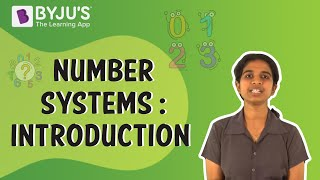## Predecessors and Successors

### Predecessor and Successor

• A successor of any number is the next number to it, which is obtained by adding 1.
• A predecessor of any number is the previous number to it, which is obtained by subtracting 1.
• For example, predecessor and successor of the number 12 is 12 – 1 and 12 + 1 which is 11 and 13

#### For More Information On Predecessors and Successors, Watch The Below Video.## Where Do Whole Numbers Live?

### Number Line

• It is the infinitely long line containing all the whole numbers.
• The line starts at zero, and any two consecutive whole numbers have the same distance between them.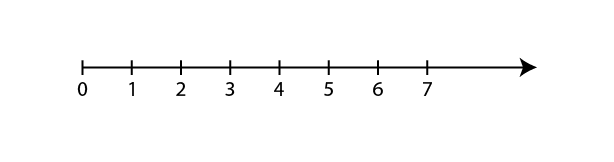To know more about Number Lines, visit here.

## Describing Number Line

### Operations on a number line

⇒ Addition on a number line. For example, addition of 1 and 5 (1 + 5 = 6). First, locate 1 on the number line. Then move 5 places to the right will give 6.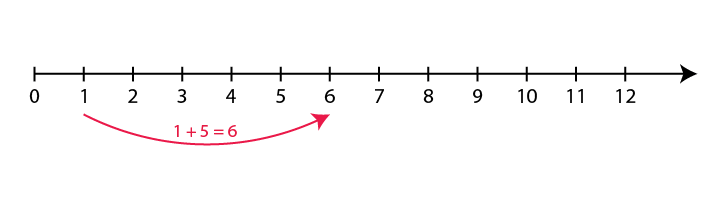⇒ Subtraction on a number line. For example, subtraction of 3 from 7 (7 – 3 = 4). First, locate 7 on the number line. Then move 3 places to the left will give 4.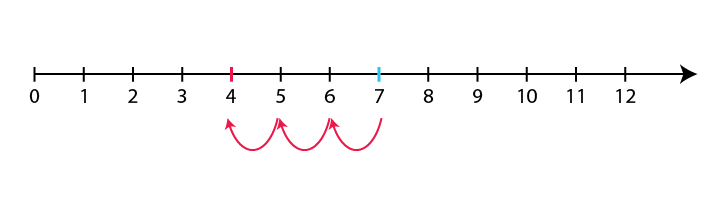⇒ Multiplication on a number line. For example product of 3 and 4 (3 × 4 = 12). Start from 0 and skip 3 places to the right 4 times.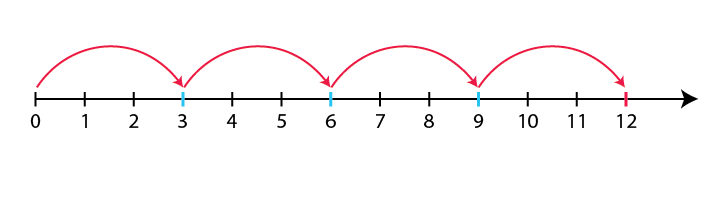⇒ Division on a number line. For example 6 ÷ 3 = 2. Start from 6 and subtract 3 for a number of times till 0 is reached. The number of times 3 is subtracted gives the quotient.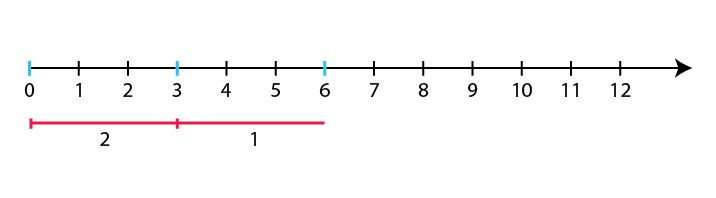## Properties of Operators: Commutative Associative and Distributive

### Division by zero

Division of any whole number by 0 is not defined.

Mathematical operations are simplified due to certain properties that every number follows. They are:

• ### Commutative property

Addition and multiplication are commutative for whole numbers. i.e whole numbers can be added or multiplied in any order.

For e.g: 2 + 3 = 5 = 3 + 3 × 4 = 12 = 4 × 3

• To know more about Commutative Property, visit here.

### Associative property

For eg: (5 +6) + 4 = 15 = 5 + (6 + 4)

(2 × 3) × 4 = 24 =2 × (3 × 4)

• To know more about Associative Property, visit here.

### Distributive Property

With distributivity property, 4 × (5 + 3) can be written as (4 × 5) + (4 × 3)

Here, 4 × (5 + 3) = 4 × 13 = 52

Also, (4 × 5) + (4 × 3) = 20 + 32 = 52

There exists certain numbers, when included in mathematical operations like addition and multiplication, the value of the operation remains unchanged. Such numbers are called as identities.
To know more about Distributive Properties, visit here.

Additive identity gives the same whole number when added to another whole number.

Zero is the additive identity as a + 0 = a, (a is any whole number).

### Multiplicative Identity

Multiplicative identity gives the same whole number when multiplied by another whole number.

1 is the Multiplicative identity as a × 1 = a, (a is any whole number)

## Let’s Play With Whole Numbers

### Patterns

• Every number can be arranged as a line.
• E.g : 5 = •••••
• Some whole numbers can be expressed as squares.
• E.g :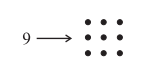• Some whole numbers can be expressed as rectangles.
• E.g : 6 can be shown as 3 × 2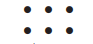• Some numbers can also be arranged as triangles.
•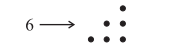### Numbers between square numbers

• Between 2 successive square numbers there exists 2n non-square numbers. Between, n² and (n + 1)² there are non-square numbers. Here is a whole number.
• For example, between 9 (3)² and 16 (4)², there are 10 , 11, 12, 13, 14, 15 which is 6 = 2 × 3 numbers.

• Sum of the first n natural odd numbers gives n² which is a perfect square.
• For example : Sum of first 5 natural odd numbers ⇒ 1 + 3 + 5 + 7 + 9 = 25 = 5²

## Properties of Operators: Closure Properties

### Closure property

Whole numbers are closed under addition and also under multiplication.

 3 + 1 = 4, a whole number 5 + 3 = 8, a whole number 4 × 4 = 16, a whole number 9 × 2 = 18, a whole number

Whole numbers are not closed under subtraction and division.

 8 – 5 = 3, a whole number 5 – 8 = -3, not a whole number 12 ÷ 4 = 3, a whole number 9 ÷ 2 = 9/2, not a whole number

## Frequently asked Questions on CBSE Class 6 Maths Notes Chapter 2: Whole Numbers

### What are ‘Whole numbers’?

A whole number is simply any positive number that does not include a fractional or decimal part.

### What are the uses of a ‘Number line’?

Number line is used as a tool to compare and order numbers and also perform various operations such as addition, subraction, etc.

### What is the ‘Additive identity’ property?

The formula of additive identity is written as a + 0 = a. This explains that when any number is added to zero, the sum is the number itself.